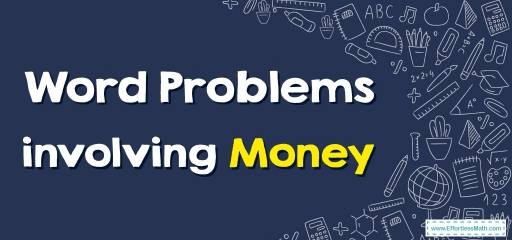# Word Problems Involving Money

Do you find it difficult to solve money word problems? Do not worry, in this article we will teach you how to solve the word problems of money with a few simple steps.Did you ever want to purchase stuff somewhere, and doubted if you had sufficient cash? If you possess sufficient cash, would there be any remaining? If so, what would you have left?

Whenever you ask those vital important questions, you’re using some essential mathematics abilities. You may estimate, or discover an answer near enough to the right one.

You’ll more than likely do one or more operations (adding, subtracting, multiplying, or dividing). You’ll utilize a lot of those abilities when you need to solve money-related problems.

## Related Topics

Whenever solving word problems, you should slow down and ask yourself a lot of questions.

Step one: Ask: What’s this problem asking me to discover? Do any clues exist?

Step two: Ask: What are the top vital words and info? Underline or circle these. Is any of the info not necessary? If so, mark it out lightly.

Step three: Set up your problem. Is it necessary to estimate? Do addition? Do subtraction? Do multiplication? Divide? Do more of these than one?

Step four: Work out your problem. Write down a number sentence. Redo your problem vertically.  Be sure the decimal points are lined up. Answer the problem and write it down in the form of dollars.

Step five: Ask: Does the answer sound logical? Is the answer sensible? If not, you should first check to see if you made any math errors. If not, ask yourself, did I perform the correct operation?

The following chart shows the common terms for the four operations that most word problems use.

### Word Problems Involving Money – Example 1:

Solve the word problem.

John has $$40.00$$. Sarah has $$17.00$$ more than John. How much is Sarah’s money?

Solution:

Sarah has $$17.00$$ more than John’s money, so Sarah’s amount of money is equal to the sum of two given numbers:$$40.00+17.00=57.00$$

### Word Problems Involving Money – Example 2:

Solve the word problem.

Lisa and Maria’s total money is $$65.00$$. If Lisa has $$35.00$$, how much does Maria have?

Solution:

The sum of Maria and Lisa’s money is equal to $$65.00$$, so subtracting Lisa’s money from the total amount of money determines the amount of Maria’s money: $$65.00-35.00=30.00$$

## Exercises for Word Problems involving Money

Solve each word problem.

1. Jonathan has $$25.00$$ and Jack has $$28.00$$. How Much Money Do Jonathan and Jack have together?
2. Michael has $$9.00$$ less than Jennifer. If Jennifer has $$20.00$$, how much money does Michael have?
3. Lara and Emma need $$80.00$$ to buy a gift for their friend. They now have a total of $$45.00$$, how little money do they have to buy the gift?
4. Angela gave Emily $$15.00$$. If she had $$28.00$$ before giving money, how much money does she have left now?
1. $$\color{blue}{53}$$
2. $$\color{blue}{11}$$
3. $$\color{blue}{35}$$
4. $$\color{blue}{13}$$

### What people say about "Word Problems Involving Money - Effortless Math: We Help Students Learn to LOVE Mathematics"?

No one replied yet.

X
30% OFF

Limited time only!

Save Over 30%

SAVE $5 It was$16.99 now it is \$11.99